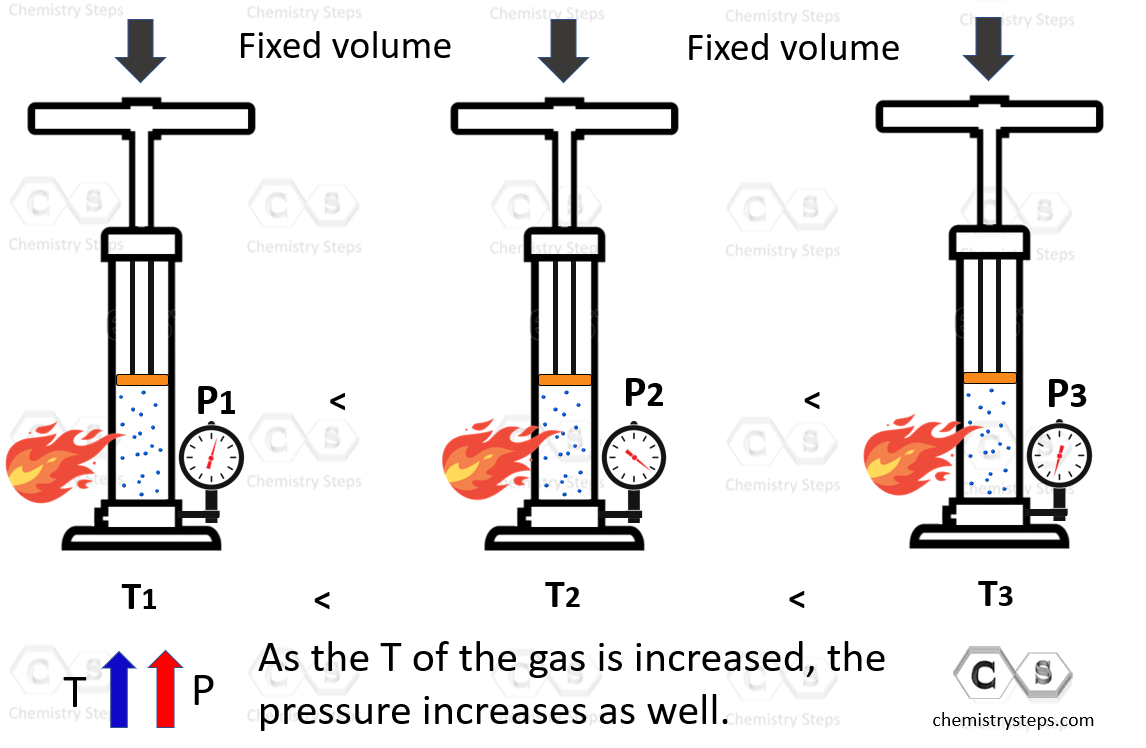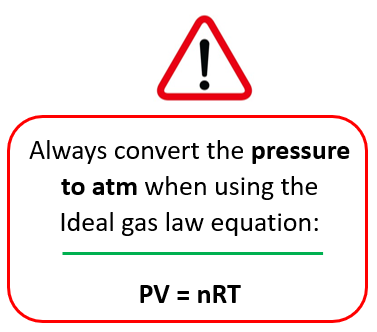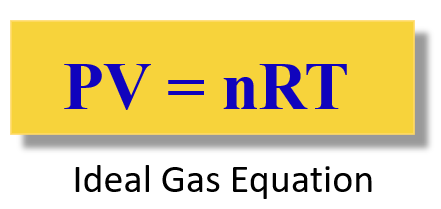## General Chemistry

The Gay-Lussac’s law demonstrates the relationship between the pressure and the temperature of an ideal gas. Let’s illustrate it by using a pump with a barrel that is held at a fixed position to prevent changing the volume. The pump is heated, and we are trying to see how increasing the temperature affects the pressure of the gas. Remember, in the experiment for the Charle’s law, heating the pump resulted in increasing the volume because the barrel could freely move. Now, here because we do not allow moving the barrel, the volume stays constant, and the pressure of the gas increases instead.The pressure is directly proportional to the temperature of the gas, so we can write that:

P ~ T

P = constant x T

This can be explained using the example of a car dealership income. The income depends on the number of sales which we can represent as:

Income  number of cars

However, we cannot say income = number of cars sold, so to switch an equal sign, we need to introduce a constant. This can be the price of the car transforming the equation to:

Income  = price x number of cars

So, for our experiment, we are not interested too much in the constant, but rather in how it links the temperature and pressure of the gas at positions 1 and 2. If we rearrange the equation with the constant, we can write that:

$\frac{{\rm{P}}}{{\rm{T}}}\;{\rm{ = }}\,{\rm{constant}}$

Because the ratio P/T is constant at any temperature and volume, we can write that:

$\frac{{{{\rm{P}}_{\rm{1}}}}}{{{{\rm{T}}_{\rm{1}}}}}\;{\rm{ = }}\,{\rm{constant}}\,{\rm{ = }}\,\frac{{{{\rm{P}}_{\rm{2}}}}}{{{{\rm{T}}_{\rm{2}}}}}$

Omitting the constant, we obtain the following equation:This is the practical implication of Gay-Lussac’s law that is used for solving gas problems.

For example,

A sample of helium gas at 1.40 atm is heated from 23.0 °C to 400.0 K. How many atmospheres is the final pressure of the helium gas?

P1 = 1.40 atm

T1 = 23.0 °C

P2 = 400.0 K

T2 = ?

Now, before doing anything else, remember to always convert the temperature to Kelvin when solving a gas problem:Convert the temperature to Kelvin right away!

T1 = 23.0 + 273 = 296 K

Write down the gas law and rearrange it to get an expression of P2.

$\frac{{{{\rm{P}}_{\rm{1}}}}}{{{{\rm{T}}_{\rm{1}}}}}\; = \;\frac{{{{\rm{P}}_{\rm{2}}}}}{{{{\rm{T}}_{\rm{2}}}}}$

${{\rm{P}}_{\rm{2}}}\; = \;\frac{{{{\rm{P}}_{\rm{1}}}{{\rm{T}}_{\rm{2}}}}}{{{{\rm{T}}_{\rm{1}}}}}\; = \;\frac{{{\rm{1}}{\rm{.40}}\;{\rm{atm}}\;{\rm{ \times }}\;{\rm{400}}{\rm{.0}}\;\cancel{{\rm{K}}}}}{{{\rm{296}}\;\cancel{{\rm{K}}}}}\;{\rm{ = }}\;{\rm{1}}{\rm{.89}}\;{\rm{atm}}$

# The Ideal Gas Law

Notice that in the experiment, we made an assumption that the pressure is constant, and this is what we also do the other gas laws to determine the desired correlation. For example, in the Boyle’s law, we study a constant amount of gas at constant temperature and find that the pressure increase as the volume is decreased.

To combine all the laws together and have the four variables (n, P, V, T) in one place, the Ideal Gas Law equation is obtained:The R is called the ideal gas constant. Although it has different values and units, you will mostly be using this:

$R\;{\rm{ = }}\;{\rm{0}}{\rm{.08206}}\;\frac{{{\rm{L}} \cdot {\rm{atm}}}}{{{\rm{mol}} \cdot {\rm{K}}}}$

The ideal gas law equation is used when you need to find P, V, T, or n, for the system where they do not change.

We will cover the ideal gas law in a separate post with some examples.

# How do I know which gas law to use?

You are probably wondering about this question now that every gas law brings a new equation. For this, there is what is called the combined gas law and as long as you remember it, you do not need to remember all the gas laws to solve a problem.

Let’s keep it for another article because there is quite a lot of information in this one.

Check Also